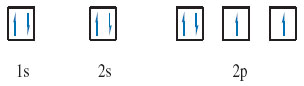Science, Maths & Technology

### Become an OU studentThe molecular world

Start this free course now. Just create an account and sign in. Enrol and complete the course for a free statement of participation or digital badge if available.

# 3.5 Electron states and box diagrams

So far, we have represented the electronic state of an atom as a collection of sub-shells. Now we turn to the states of the electrons within those sub-shells. Just as shells can be broken down into sub-shells, so sub-shells can be broken down into atomic orbitals. Each atomic orbital describes an allowed spatial distribution about the nucleus for an electron in the sub-shell. Here we shall only be concerned with their number.

Consider the formula for the sub-shell electron capacities, which is 2(2l + 1), l being the second quantum number. The factor (2l + 1) tells us the number of atomic orbitals in the sub-shell.

## Question 18

How many atomic orbitals are there in an s sub-shell, and how many in a p sub-shell?

One and three, respectively; for s and p sub-shells, l = 0 and 1, so (2l + 1) = 1 and 3, respectively.

It turns out that each orbital in a sub-shell can contain up to two electrons. This is connected to a property of the electron called spin. This spin occurs in one of two senses, which are physically pictured as clockwise and anticlockwise (Figure 25).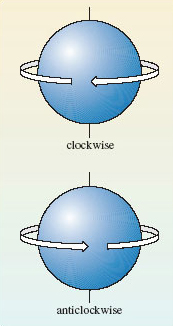Figure 25 The two senses of electron spin.

When an atomic orbital contains its maximum complement of two electrons, those two electrons must always have spins of opposite sense. This phenomenon of electron spin accounts for the '2' that precedes the bracket in the formula for sub-shell capacities, 2(2l + 1). For example, as we have seen, the factor (l + 1) tells us that there are three atomic orbitals in a p sub-shell. Each of the three orbitals can accommodate up to two electrons with opposed spins. So a p sub-shell can contain a maximum of 2 × 3 or 6 electrons.

A full atomic orbital, therefore, contains two electrons with spins of opposite sense. This is represented by writing one electron as an upward-pointing half-headed arrow, and the other as a downward-pointing half-headed arrow. For example, the helium atom has the electronic configuration 1s2. The 1s sub-shell contains just one orbital, which can be represented by a single box containing the two electrons of opposite spin: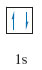Two such electrons with opposed spins are said to be paired and the diagram that puts these electrons into boxes is referred to as a box diagram. Now consider the case of nitrogen, whose atom has the configuration 1﻿s﻿2﻿2﻿s﻿2﻿2﻿p﻿3. The 1s and 2s sub-shells contain one orbital each, and the 2p sub-shell contains three. The box diagram that must be filled therefore takes the form: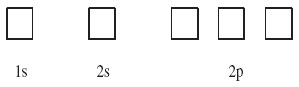## Question 19

Assign the two 1s and two 2s electrons of nitrogen to boxes in this diagram.

The 1s and 2s boxes should now both look like the 1s box for the helium atom above: they should each contain two electrons with opposed spins.

The final step is the assignment of the three 2p electrons to the three 2p boxes. There are several possibilities, but the one we want is the ground-state arrangement, the state of lowest energy. There is a simple rule, called Hund's rule, that tells us what this is:

Within any sub-shell, there will be the maximum number of electrons with spins of the same sense.

Because electrons in the same box must have opposed spins, we must put the electrons, as far as possible, in different boxes with spins of the same sense, or, as it is usually termed, with parallel spins. In this case there are three 2p electrons and three boxes, so each box can take one electron with the same spin, and this is the preferred arrangement according to Hund's rule. The final result for the nitrogen atom is therefore: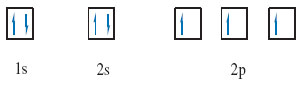## Question 19a

The oxygen atom, with the configuration 1s22s22p4, has one more electron than the nitrogen atom. Draw the box diagram for the oxygen atom.